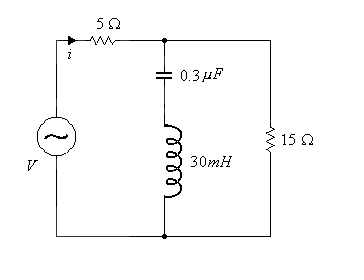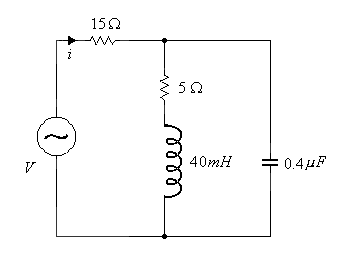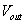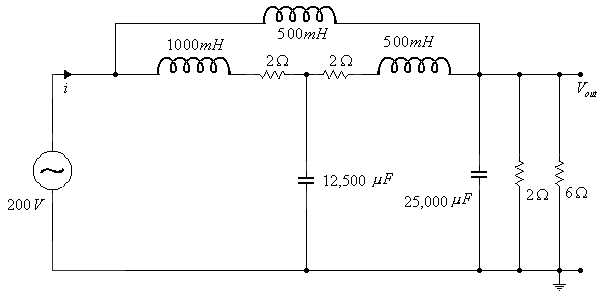EL10A TUTORIAL #3   Sunday, 9 March 2003

1. (a) At what frequency will the main current i be in phase with the voltage V ?
(b) If V=200 Volts what will be the value of i at the frequency you calculated in (a) ?2. (a) At what frequency will the main current i be in phase with the voltage V ?
(b) If V=150 Volts what will be the value of i at the frequency you calculated in (a) ?3. (a) Find i for 50Hz and 100Hz and give the power factor in both cases
(b) Findfor the 100Hz case only